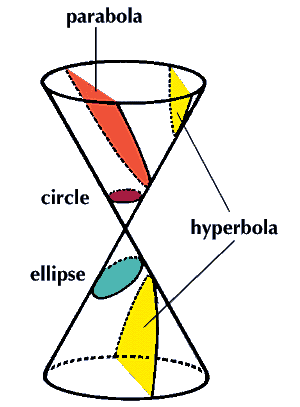#Conic Sections

Math Lair Home > Topics > Conic SectionsThe first person to describe conic sections in the formal language of Euclid's geometry, as well as coining the names of three of the conic sections, was the ancient Greek mathematician Appolonius of Perga. The four conic sections are the circle, the ellipse, the parabola, and the hyperbola (the last three names being the ones coined by Appolonius). They are called conic sections because they are the shapes formed by the intersection of a plane with a conical surface. As well, the general class of conic sections also includes as special cases a point (at the cone's apex) and pairs of straight lines (degenerate hyperbolas passing through the apex of the cone). The type of conic section produced by this intersection depends on the angle at which the plane intersects the cone's surface.

A circle is defined as the set of points that are equidistant from a point. A variation of this would be to find all points for which the distance from that point to a reference point is equal to the distance from that point to a line multiplied by a constant. This technique is so important that special names are used for the line, the point, and the constant. The point is the focus. The line is called the directrix. The constant, which is defined as the ratio of the distance from the focus to the distance from the directrix is the eccentricity, which is abbreviated as e.

A circle is defined to have eccentricity 0. Every eccentricity between (but not including) 0 and 1 is an ellipse. If e = 1, the curve produced is a parabola. Every point on a parabola is equidistant from the focus and the directrix. Unlike an ellipse, notice that a parabola is not a closed curve. Curves with eccentricity greater than 1 are called hyperbolas. Notice that they include points on both sides of the directrix.

Also, a conic section is produced when an equation of the second degree is graphed. An equation of the second degree is one of the form

ax2 + bxy + cy2 + dx + ey + f = 0

where a through f are constants. For example:

• A circle is obtained when b = 0 and a = c.
• An ellipse whose axes are aligned with the axes of the graph is produced when b = 0 and ac is positive and not equal to 1.
• A parabola is obtained when b = 0 and one of a or c is equal to 0.
• A hyperbola whose axes are aligned with the axes of the graph is produced when b = 0 and ac is negative.